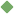statistics 1. [ noun ] (mathematics,statistics) a branch of applied mathematics concerned with the collection and interpretation of quantitative data and the use of probability theory to estimate population parameters Related terms: applied_mathematics least_squares biometrics multiple_regression regression regression_analysis nonparametric_statistics correlational_analysis time_series correlation distribution information_theory statistical_method average distribution average sample_distribution mean binomial_distribution standard_deviation statistic sampling second_moment significant universe correlation_coefficient modal grab_sample regression_line random_sample median degree_of_freedom normal_distribution normal_curve deviation arithmetic_mean weight regression_equation correlation_matrix harmonic_mean geometric_mean partial_correlation variance Bernoulli's_law dependent_variable negative_correlation multiple_correlation_coefficient covariance rank-order_correlation_coefficient nonparametric_statistic least_squares stochastic coefficient_of_concordance tau_coefficient_of_correlation Kendall_partial_rank_correlation split-half_correlation spurious_correlation Poisson_distribution frequency_distribution binomial_theorem subpopulation information_theory centile correlation_table random_sample curvilinear_correlation parametric_statistic first-order_correlation ergodic positive_correlation supernormal product-moment_correlation_coefficient quartile biserial_correlation_coefficient regression stratified_sample analysis_of_variance Kendall_test experimental_variable multiple_regression outlier phi_coefficient predictor_variable tetrachoric_correlation_coefficient regression_analysis demographic mean_deviation multicollinearity statistician probability_theory mode multivariate nonsignificant decile regression_coefficient statistical_method linear_regression vital_statistics median cross_section curvilinear_regression moment nonparametric bayes'_theorem bayes'_postulate multivariate_analysis correlational_analysis factor_analysis time_series subnormal unimodal bimodal To share this definition press "text" (Facebook, Twitter) or "link" (blog, mail) then paste text link Similar spelling: statistic   statistical   statistician   statistically   statics   statice   Studstill   statically